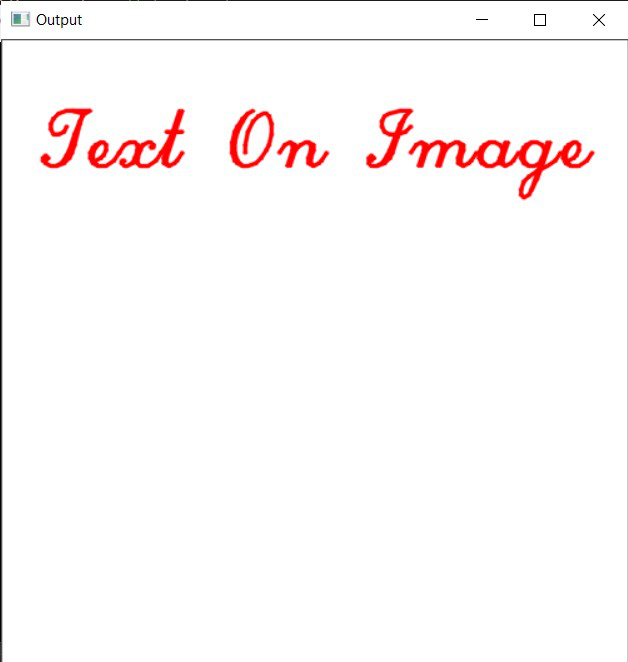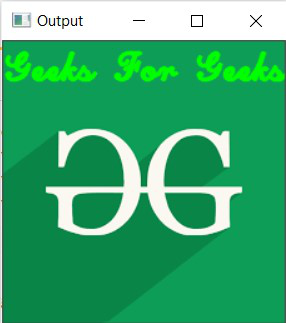Related Articles
Write on an image using openCV in C++
• Last Updated : 04 Feb, 2021

In this article, we will discuss how to write over an image using OpenCV C++. Function putText() from OpenCV C++ library will be used to write text on an image.

Program 1:

Below program shows how to write text over a blank background image:

## C++

 `// C++ program to demonstrate the``// above approach``#include ``#include `` ` `// Library to include for``// drawing shapes``#include ``#include ``using` `namespace` `cv;``using` `namespace` `std;`` ` `// Driver Code``int` `main(``int` `argc, ``char``** argv)``{``    ``// Create a blank image of size``    ``// (500 x 500) with white background``    ``// (B, G, R) : (255, 255, 255)``    ``Mat image(500, 500, CV_8UC3,``              ``Scalar(255, 255, 255));`` ` `    ``// Check if the image is created``    ``// successfully.``    ``if` `(!image.data) {``        ``cout << ``"Could not open or"``             ``<< ``" find the image"``             ``<< endl;``        ``return` `0;``    ``}`` ` `    ``// Writing over the Image``    ``Point org(30, 100);``    ``putText(image, ``"Text On Image"``, org,``            ``FONT_HERSHEY_SCRIPT_COMPLEX, 2.1,``            ``Scalar(0, 0, 255), 2, LINE_AA);`` ` `    ``// Show our image inside a window.``    ``imshow(``"Output"``, image);``    ``waitKey(0);`` ` `    ``return` `0;``}`

Output:Program 2:

Below program shows how to write text over a loaded image:

## C++

 `// C++ program to demonstrate the``// above approach``#include ``#include `` ` `// Library to include for``// drawing shapes``#include ``#include ``using` `namespace` `cv;``using` `namespace` `std;`` ` `// Driver Code``int` `main(``int` `argc, ``char``** argv)``{``    ``// Create a blank image of size``    ``// (500 x 500) with white background``    ``// (B, G, R) : (255, 255, 255)``    ``Mat image = imread(``"C:/Users/harsh/Downloads/geeks.png"``,``                       ``IMREAD_COLOR);`` ` `    ``// Check if the image is``    ``// created successfully.``    ``if` `(!image.data) {``        ``cout << ``"Could not open or"``             ``<< ``" find the image"` `<< std::endl;``        ``return` `0;``    ``}`` ` `    ``// Writing over the Image``    ``Point org(1, 30);``    ``putText(image, ``"Geeks For Geeks"``, org,``            ``FONT_HERSHEY_SCRIPT_COMPLEX, 1.0,``            ``Scalar(0, 255, 0), 2, LINE_AA);`` ` `    ``// Show our image inside a window.``    ``imshow(``"Output"``, image);``    ``waitKey(0);`` ` `    ``return` `0;``}`

Output:Want to learn from the best curated videos and practice problems, check out the C++ Foundation Course for Basic to Advanced C++ and C++ STL Course for foundation plus STL.  To complete your preparation from learning a language to DS Algo and many more,  please refer Complete Interview Preparation Course.
My Personal Notes arrow_drop_up